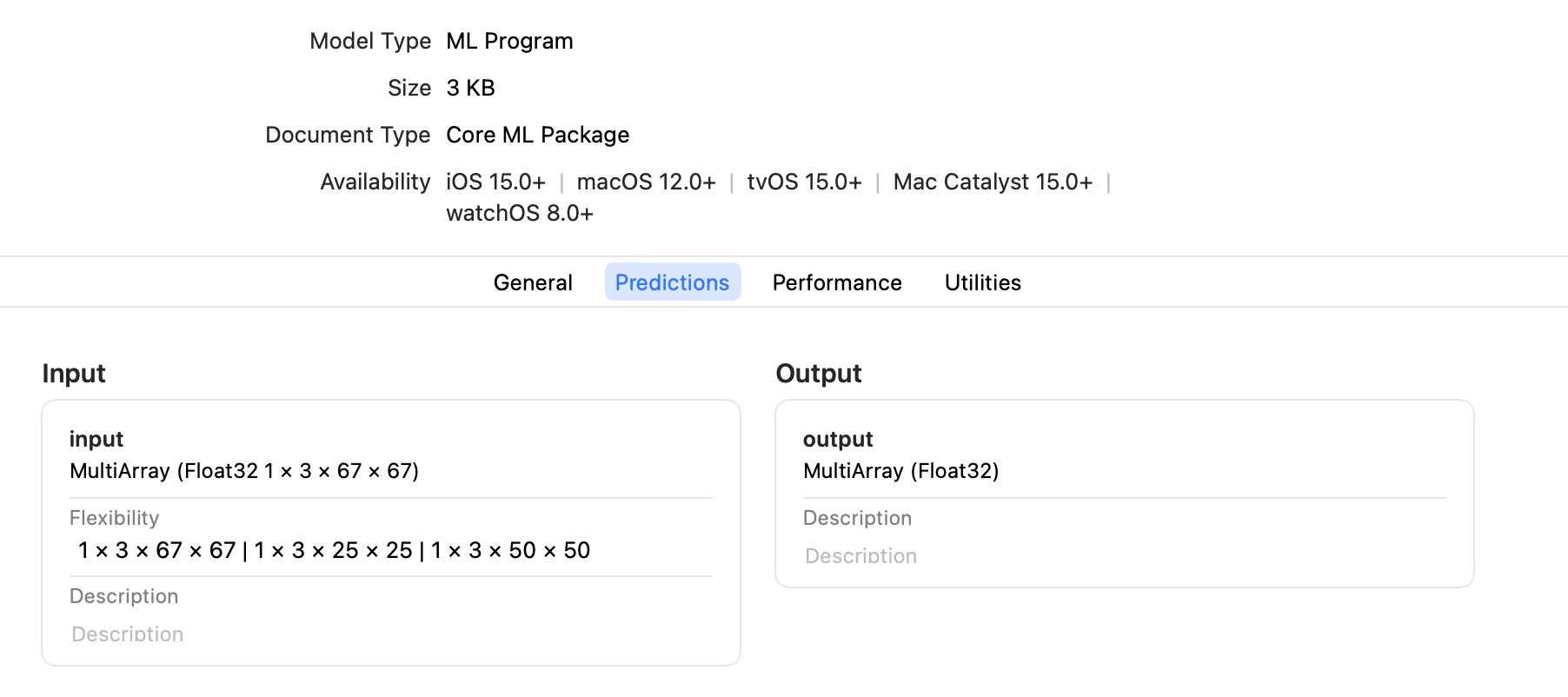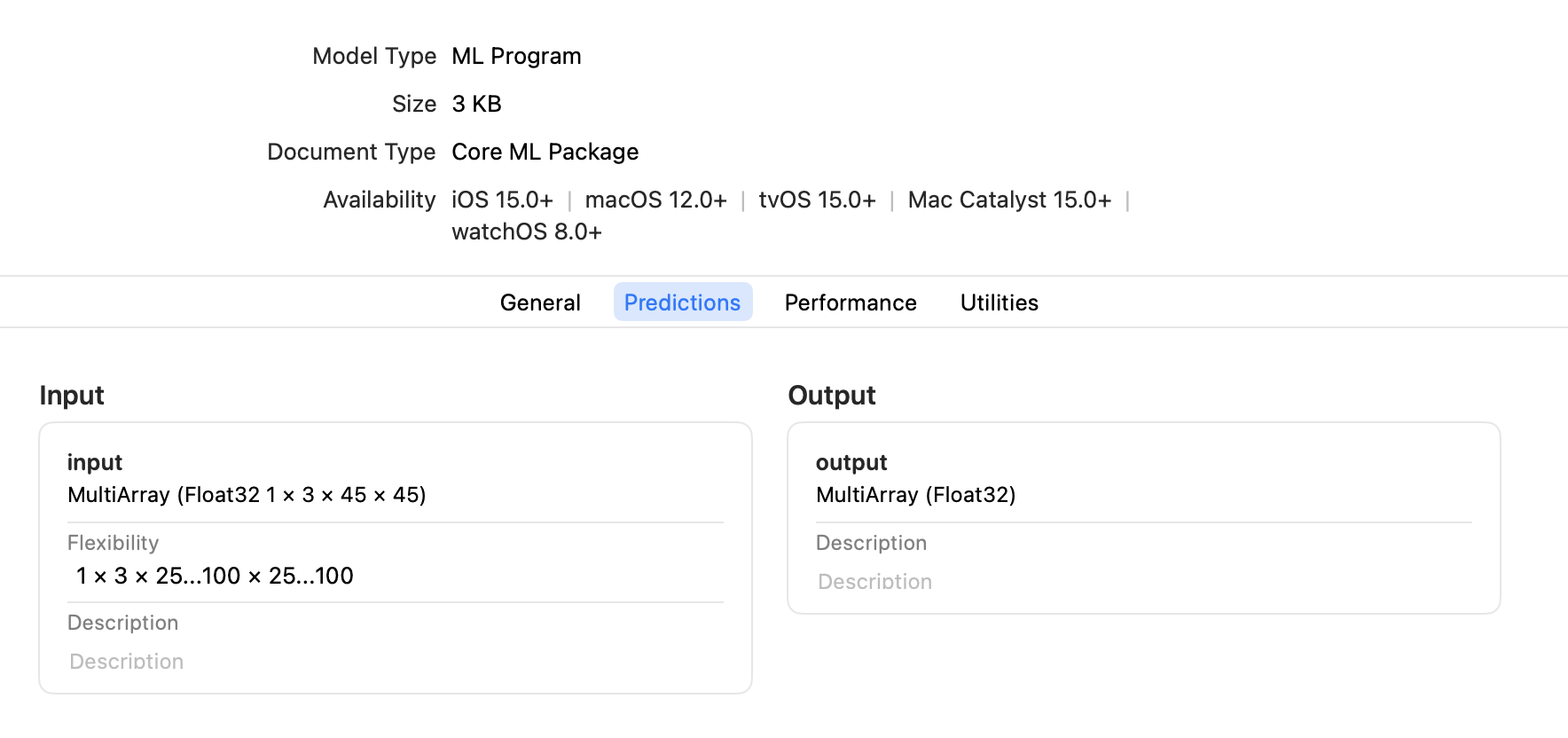## coremltools

Use Core ML to integrate machine learning models into your app. Core ML provides a unified representation for all models. Your app uses Core ML APIs and user data to make predictions, and to train or fine-tune models, all on the user’s device.

Core ML optimizes on-device performance by leveraging the CPU, GPU, and Neural Engine while minimizing its memory footprint and power consumption. Running a model strictly on the user’s device removes any need for a network connection, which helps keep the user’s data private and your app responsive.

# Flexible Input Shapes

While some neural network models accept only fixed-size input, such as an image with a resolution of 224 x 224 pixels, other models require flexible input shapes that are determined at runtime. Examples are language models that work on arbitrary input lengths, and style transfer models that work on multiple resolutions.

When converting a model to Core ML using Core ML Tools, you can specify a fixed input shape or a flexible input shape. With a flexible input shape you can specify the input as follows:

## Select From Predetermined Shapes

To optimize performance, ensure model accuracy, or limit the range of inputs for other purposes, specify a set of predetermined shapes for input. To make Core ML models using this constraint, set EnumeratedShapes as shown in the following example.

### 👍Enumerated Shapes Provide a Performance Advantage

Use EnumeratedShapes for best performance. During compilation the model can be optimized on the device for the finite set of input shapes. You can provide up to 128 different shapes. If you need more flexibility for inputs, consider setting the range for each dimension.

The following example performs these steps:

1. Defines the model TestConvModule using torch.nn.Module, the base class for neural network modules, and traces the model with random input.
2. Defines the input_shape with EnumeratedShapes. You can optionally specify a default shape, or set default to None (or omit default) to use the first element in shapes as the default. The default shape loads when initiating the model.
3. Converts the model using input_shape to an ML program and saves it as an ML package.
4. Tests the model with predictions.
import coremltools as ct
import torch
import numpy as np

# Define a model for this example.
class TestConvModule(torch.nn.Module):
def __init__(self, in_channels=3, out_channels=10, kernel_size=3):
super(TestConvModule, self).__init__()
self.conv = torch.nn.Conv2d(in_channels, out_channels,
kernel_size)

def forward(self, x):
return self.conv(x)

# Trace the model with random input.
example_input = torch.rand(1, 3, 50, 50)
traced_model = torch.jit.trace(TestConvModule().eval(), example_input)

# Set the input_shape to use EnumeratedShapes.
input_shape = ct.EnumeratedShapes(shapes=[[1, 3, 25, 25],
[1, 3, 50, 50],
[1, 3, 67, 67]],
default=[1, 3, 67, 67])

# Convert the model with input_shape.
model = ct.convert(traced_model,
inputs=[ct.TensorType(shape=input_shape, name="input")],
outputs=[ct.TensorType(name="output")],
convert_to="mlprogram",
)

model.save("enumerated_shapes.mlpackage")

# Test the model with predictions.
input_1 = np.random.rand(1, 3, 25, 25)
input_2 = np.random.rand(1, 3, 67, 67)

output_1 = model.predict({"input": input_1})["output"]
print("output shape {} for input shape {}".format(output_1.shape, input_1.shape))
output_2 = model.predict({"input": input_2})["output"]
print("output shape {} for input shape {}".format(output_2.shape, input_2.shape))


You can open the saved ML package in Xcode and click the Predictions tab to display the input and output, as shown in the following figure.### 📘Core ML Preallocates the Default Shape

Core ML preallocates the memory for the default shape, so the first prediction with the default shape is fast. The first prediction with a non-default shape may be slower, but subsequent predictions should be more optimized.

### 📘Enumerated Shapes with Multi-input Models

For a multi-input model, only one of the inputs can be marked with EnumeratedShapes; the rest must have fixed single shapes. If you require multiple inputs to be flexible, set the range for each dimension.

## Set the Range for Each Dimension

If you know that the input shape will be within a specific interval in each dimension, set the range for each dimension with RangeDim as shown in the following example. Using a bounded range for each dimension provides more opportunity for the runtime compiler to make optimizations, which is harder to do with an unbounded range.

The code in the following example performs these steps:

1. Defines the model TestConvModule, and traces the model with random input.
2. Defines the input_shape with RangeDim. You can optionally specify a default shape, or set default to None (or omit default) to use the lower_bound as the default.
3. Converts the model using input_shape to an ML program and saves it as an ML package.
4. Tests the model with predictions.
import coremltools as ct
import torch
import numpy as np

# Define a model for this example.
class TestConvModule(torch.nn.Module):
def __init__(self, in_channels=3, out_channels=10, kernel_size=3):
super(TestConvModule, self).__init__()
self.conv = torch.nn.Conv2d(in_channels, out_channels,
kernel_size)

def forward(self, x):
return self.conv(x)

# Trace the model with random input.
example_input = torch.rand(1, 3, 50, 50)
traced_model = torch.jit.trace(TestConvModule().eval(), example_input)

# Set the input_shape to use RangeDim for each dimension.
input_shape = ct.Shape(shape=(1,
3,
ct.RangeDim(lower_bound=25, upper_bound=100, default=45),
ct.RangeDim(lower_bound=25, upper_bound=100, default=45)))

# Convert the model with input_shape.
model = ct.convert(traced_model,
inputs=[ct.TensorType(shape=input_shape, name="input")],
outputs=[ct.TensorType(name="output")],
convert_to="mlprogram",
)

model.save("range_shapes.mlpackage")

# Test the model with predictions.
input_1 = np.random.rand(1, 3, 25, 25)
input_2 = np.random.rand(1, 3, 67, 67)

output_1 = model.predict({"input": input_1})["output"]
print("output shape {} for input shape {}".format(output_1.shape, input_1.shape))
output_2 = model.predict({"input": input_2})["output"]
print("output shape {} for input shape {}".format(output_2.shape, input_2.shape))


You can open the saved ML package in Xcode and click the Predictions tab to display the input and output, as shown in the following figure.## Enable Unbounded Ranges

To enable an unbounded range, which allows the input to be as large as needed, set the upper_bound with RangeDim to -1 for no upper limit. However, the device's memory constraints actually determine how large the input can be. Set a maximum range for upper_bound, as shown in the previous example, to ensure that the model doesn't crash at runtime for your users.

## Update a Core ML Model to Use Flexible Input Shapes

To update a model with fixed input shapes to use flexible input shapes, the best practice is to specify a flexible input shape when converting the source model to Core ML, as described in the previous sections.

However, if the source model is not available, you can still update an existing Core ML model with fixed input shapes to use flexible input shapes. Use the neural_network.flexible_shape_utils, which is a set of utilities to annotate model input/output features with flexible shape information.

### 🚧Available Only for Neural Networks

The neural_network.flexible_shape_utils update the model's specification, but do not guarantee the model's accuracy with flexible input shapes. These utilities are available only for a neuralnetwork, not for an mlprogram.

The following code snippet shows how to update model inputs using add_multiarray_ndshape_enumeration() and set_multiarray_ndshape_range():

# Enumerated shapes and multi array inputs

from coremltools.models.neural_network import flexible_shape_utils

# to update the shapes of the first input
input_name = spec.description.input.name

feature_name=input_name,
enumerated_shapes=[(2,4), (2,6)])

# Range shapes and multi array inputs
# -1 in upper bound represents "infinity"
flexible_shape_utils.set_multiarray_ndshape_range(spec,
feature_name=input_name,
lower_bounds=[1,2],
upper_bounds=[10,-1])

# re-initialize the mlmodel object
model = coremltools.models.MLModel("mymodel_updated.mlmodel")


## API Reference

For details, see the following API documentation:Updated 6 months ago

# Flexible Input Shapes

### Suggested Edits are limited on API Reference Pages

You can only suggest edits to Markdown body content, but not to the API spec.